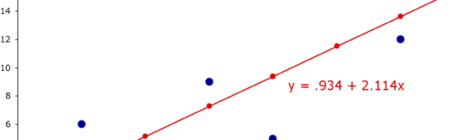## Solution assignment01 of the basics of Statistics## Regression

Simple linear regression models In the introduction to regression we discussed the (univariate) linear model: where   and are unknown population parameters which have to...